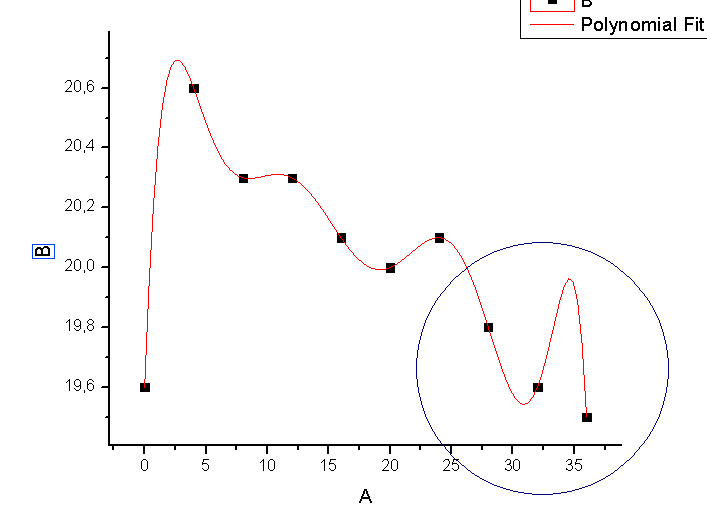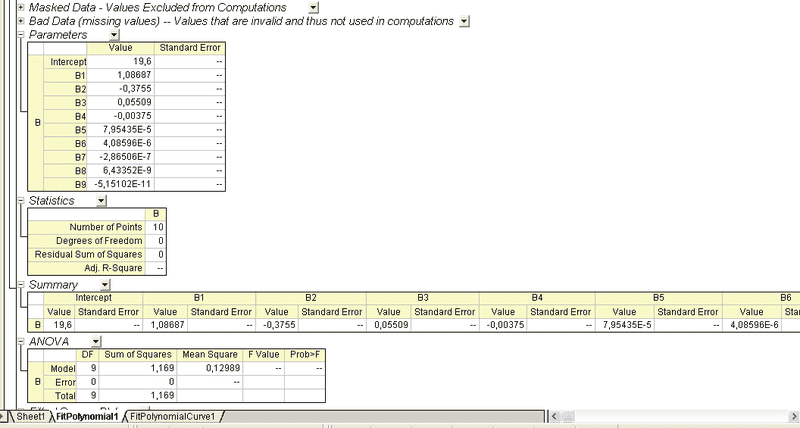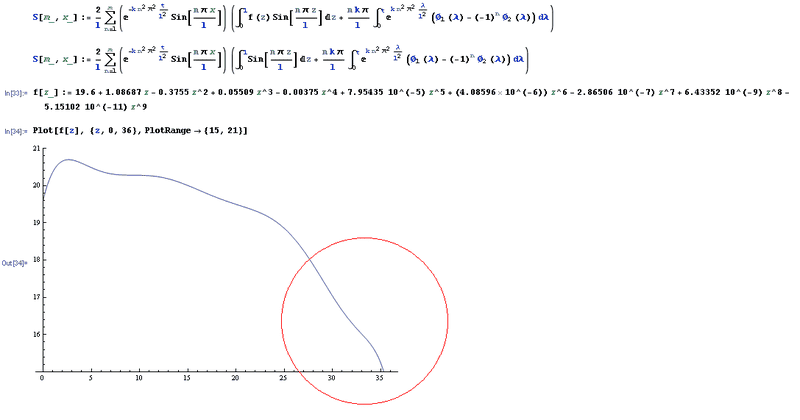# Origin fit plotted on mathematica

• Mathematica
• Telemachus
In summary: Anyway, thanks for trying to help.In summary, Bill provided the values for z in Originlab and Origin gave him back a polynomial fit. The polynomial plot on Originlab does not match the plot on mathematica.

#### Telemachus

Hi there. I have a problem with this. I'm trying to plot a polynomial fit done with origin 8.5 on mathematica. The thing is that the polynomial plot I get in mathematica from the coefficients given in originlab don't match, and I don't know why. I'll let you some pics of what I'm trying to do, the first one is the fit on origin lab, the second the tabs with the coefficients, and the third the plot on mathematica. I also draw two circles in the part of the graph which makes clear the differences between one plot and the other.

What could be happening? is that the software isn't working or did I made any mistake when going from origin to mathematica?

I tried to replace the exponential basis from 10 to e, but that makes it look even worse.

Img. 1Img. 2Img. 3Bye there, and thank you in advance.

#### Attachments

Would you consider posting as plain ordinary ascii text the list of (x,y) pairs you gave Origin and the polynomial coefficients that Origin gave you back? Literally scrape the exact values off the screen or in some other way provide the numbers in text form.

With plain ordinary ascii text anyone trying to reproduce the problem and offer a solution isn't required to type the whole thing back in, and even then can't even be sure they are using the exact same data.

Hi Bill, thanks for posting. I'm sorry I couldn't answer you on yestarday. But here it is.

Value
B Intercept...19,6
B B1.....1,08687
B B2.....-0,3755
B B3.....0,05509
B B4.....-0,00375
B B5.....7,95435E-5
B B6.....4,08596E-6
B B7.....-2,86506E-7
B B8.....6,43352E-9
B B9.....-5,15102E-11

I just copied it from the table that you can see in Img. 2. And here I give you the code I tiped into mathematica when plotting the polynomial:

f[z_] := 19.6 + 1.08687*z - 0.3755 z^2 + 0.05509*z^3 - 0.00375*z^4 +
7.95435 10^(-5)*z^5 + (4.08596 10^(-6))*z^6 - 2.86506 10^(-7)*z^7 +
6.43352 10^(-9)*z^8 - 5.15102 10^(-11)*z^9

I made the fit entirely with mathematica, and now it looks like the one given by origin. Perhaps the values origin gives in the table are truncated.

## 1. What is Origin fit plotted on Mathematica?

Origin fit plotted on Mathematica is a tool used for data analysis and visualization. It combines the powerful curve fitting capabilities of Origin with the advanced plotting and graphing features of Mathematica, allowing for more comprehensive analysis of data.

## 2. How is Origin fit plotted on Mathematica different from other data analysis tools?

Origin fit plotted on Mathematica stands out from other data analysis tools because it combines the strengths of two powerful software programs. Origin is known for its robust curve fitting capabilities, while Mathematica is known for its advanced plotting and graphing features. By combining these strengths, Origin fit plotted on Mathematica offers a more comprehensive and efficient data analysis experience.

## 3. Can Origin fit plotted on Mathematica handle large datasets?

Yes, Origin fit plotted on Mathematica can handle large datasets. It has the ability to process and analyze large amounts of data with ease and can handle datasets with millions of data points.

## 4. Is Origin fit plotted on Mathematica user-friendly?

Origin fit plotted on Mathematica is designed to be user-friendly, with a user-friendly interface and intuitive tools and features. It also offers tutorials and online resources to help users get started and make the most out of the software.

## 5. How can Origin fit plotted on Mathematica help in scientific research?

Origin fit plotted on Mathematica can aid in scientific research by providing powerful tools for data analysis and visualization. It allows for the creation of high-quality graphs and charts, making it easier to interpret and present data. It also has advanced curve fitting capabilities, which can be useful in analyzing complex relationships between variables in scientific research.